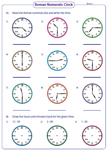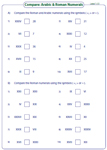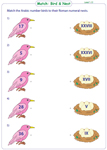1. Worksheets>
2. Math>
3. Number Sense>
4. Roman Numerals

# Roman Numerals Worksheets

Roman numerals worksheets contain abundant skills on converting Arabic numbers into Roman numerals, reading and writing the time from a Roman clock, completing the pattern, comparing and ordering the Roman numerals and adding and subtracting them. Also interesting cut paste activities are included for 1st grade, 2nd grade, and 3rd grade children. Try some of these worksheets for free!

Printable Charts

This page contains Roman numeral display charts, blank charts and partially filled charts. Beautiful themes like warrior, armor and shield are used.

Roman Numeral Charts

Arabic and Roman Numerals

There are nine different worksheet pdfs for practice in this section. In section A, write the Roman numeral for each Arabic number and vice-versa in section B.Level 1:

Level 2:

Level 3:

Roman Clock

In section A, read the Roman numeral clock and write the time in Arabic numbers. In section B, draw the minutes hand and hours hand on the Roman numeral clock. This Roman clock worksheets are ideal for grade 1 and grade 2.Complete the pattern

Complete the pattern by filling in the missing Roman numerals. For a better understanding of Roman number concept, complete the number line.Level 1:

Level 2:

Comparing Numbers: Cut-paste activity

Use the large alligator, little bird and crazy frog in these six printable activity based worksheets for grade 2 and grade 3 to compare the Roman numerals.Level 1:

Level 2:

Compare using symbols

Nine pdf worksheets spread across three levels are given here to compare the Roman numerals using the symbols <, > or =.Level 1:

Level 2:

Level 3:

Match the numbers

Three distinctive themes are used in these worksheets. Match each Arabic bird, lion and fish to its Roman numeral nest, den and pot respectively.Level 1:

Level 2:

Order the Roman numerals

Order the Roman numerals either in increasing or decreasing order. Numbers used in Level 1 are from 1-39 and level 2 are 1-399. Convert Roman numerals into Arabic numbers for better understanding.Level 1:

Level 2:

Adding and Subtracting Roman numerals

Each printable worksheet has four exclusive sections for practice. Add or subtract the Arabic numbers and Roman numerals in these worksheets.Level 1:

Level 2:

Input-Output Machine

Arabic number inputs are given through an in-out machine. Write the Roman numeral output for the given input.Level 1:

Level 2:

Related Worksheets